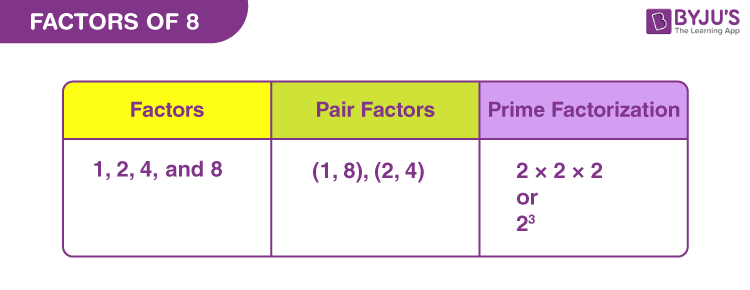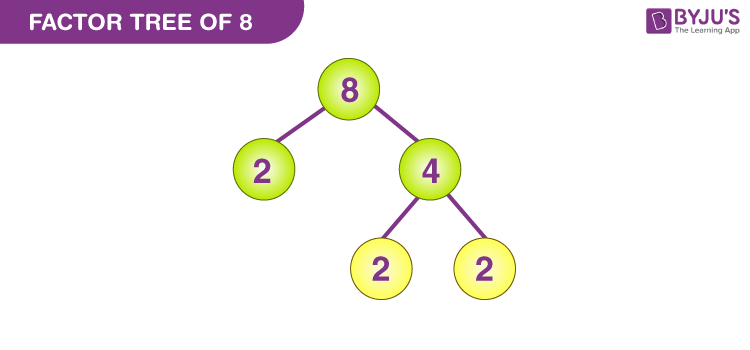# Factors of 8

The factors of 8 are the numbers that divide 8 exactly without leaving any remainder. There are four factors of 8, they are 1, 2, 4, and 8. Hence, the highest factor is 8 which divides itself. If we add all the factors, then the sum will be equal to 15.

The pair factors of 8 are (1, 8) and (2, 4). These pair factors when multiplied together result in the original number. 8 is also a perfect cube. We can determine the factors of 8 by the division method and prime factorisation method.

## What are the Factors of 8?

A number or an integer that divides 8 exactly without leaving a remainder, then the number is a factor of 8. As the number 8 is an even composite number, it has more than two factors. Thus, the factors of 8 are 1, 2, 4 and 8.## Pair Factors of 8

A pair of numbers, which are multiplied together resulting in an original number 8 is called the pair factors of 8. As discussed above, the pair factors of 8 can be positive or negative. The positive and negative pair factors of 8 are given below:

Positive Pair Factors of 8:

 Positive Factors of 8 Positive Pair Factors of 8 1 × 8 (1, 8) 2 × 4 (2, 4)

Negative Pair Factors of 8:

 Negative Factors of 8 Negative Pair Factors of 8 -1 × -8 (-1, -8) -2 × -4 (-2, -4)

## How to Find Factors of 8?

8 is a whole number that is also an even number. Since it is a single-digit number, therefore, it is much easier to find the factors of 8.

There are two methods to find the factors of 8:

• Division method
• Prime factorisation method

## Factors of 8 by Division Method

The factors of 8 can be found using the division method. In the division method, we need to find the integers which divide 8 exactly without leaving a remainder, and those integers are considered as the factors of 8. Now, let us find the factors of 8 using the division method.

• 8/1 = 8 (Factor is 1 and Remainder is 0)
• 8/2 = 4 (Factor is 2 and Remainder is 0)
• 8/4 = 2 (Factor is 4 and Remainder is 0)
• 8/8 = 1 (Factor is 8 and Remainder is 0)

If we divide 8 by any numbers other than 1, 2, 8 and 8, it leaves a remainder. Hence, the factors of 8 are 1, 2, 4 and 8.

## Prime Factorization of 8

The prime factorization of 8 is the process of writing the number as the product of its prime factors. Now, let us discuss the process of finding the prime factors of 8.

• Divide 8 by the smallest prime number, i.e. 2, i.e., 8/2 = 4.
• Divide 4 by the smallest possible prime number, i.e., 4/2 = 2.
• 2 is itself a prime number and is divisible by 2.
• Therefore, the prime factorization of 8 is 2 × 2 × 2 or 23.

## Video Lesson on Prime FactorsAnother method to find the prime factors of 8 is the factor tree method.

### Factor TreeHence, the number of unique prime factors of 8 is one. The prime factorisation of the whole number 8 is 23. The exponent in the prime factorisation is 3. When you add the number 1 with the exponent, i.e., 3 +1 = 4. Therefore, the number 8 has 4 factors.

## Facts – Factors of 8

• Factors of 8: 1, 2, 4 and 8
• Prime factorisation: 2 ×× 2
• Prime factor of 8: 2
• Pair factors: (1, 8) and (2, 4)
• Sum of factors of 8: 15

### Related Articles

 Links Related to Factors Factors of 15 Factor of 36 Factors of 48 Factors of 18 Factors of 24 Factors of 25 Factors of 42 Factors of 60 Factors of 35 Factors of 81 Factors of 75 Factors of 56

## Solved Examples on Factors of 8

Example 1:

Find the common factors of 8 and 5.

Solution:

The factors of 8 are 1, 2, 4 and 8.

The factors of 5 are 1 and 5.

As 5 is a prime number, the common factor of 8 and 5 is 1.

Example 2:

Find the common factors of 8 and 9.

Solution:

Factors of 8 = 1, 2, 4 and 8.

Factors of 9 = 1, 3, and 9.

Hence, the common factor of 8 and 9 is 1.

Example 3:

Find the common factors of 8 and 4.

Solution:

The factors of 8 are 1, 2, 4 and 8.

The factors of 4 are 1, 2 and 4.

Hence, the common factors of 8 and 4 are 1, 2, and 4.

### Practise Questions on Factors of 8

1. What is the lowest factor of 8?
2. Is there any odd number that is a factor of 8?
3. How many even numbers are the factors of 8?

Stay tuned with BYJU’S to know about factor 8 and the factors and prime factors of other numbers. Download BYJU’S – The Learning App to better experience and clarification.

## Frequently Asked Questions on Factors of 8

Q1

### What are the factors of 8?

The factors of 8 are 1, 2, 4 and 8.

Q2

### What is the prime factorization of 8?

The prime factorization of 8 is 2 × 2 × 2 or 23.

Q3

### What is the sum of all factors of 8?

The sum of all factors of 8 is 15.
Q4

### What is the greatest common factor of 8 and 7?

The greatest common factor of 8 and 7 is 1.
Q5

### What are the positive pair factors of 8?

The positive pair factors of 8 are (1, 8) and (2, 4).

Q6

### Write down the negative pair factors of 8.

The negative pair factors of 8 are (-1, -8) and (-2, -4).

Q7

### Is 2 a factor of 8?

Yes, 2 is a factor of 8. If 8 is divisible by 2, it leaves a quotient of 4 and remainder 0, and hence 2 is a factor of 8.

Test your knowledge on Factors of 8